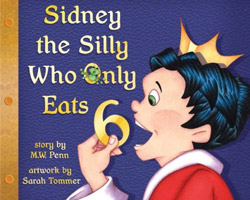# Lesson Plan for Sidney the Silly (dividing)

A Lesson Plan for Sidney the Silly (dividing)Sidney the Silly Who Only Eats 6
The theme of Sidney is number sense and problem solving.

CCSS

3.NF.1. Understand a fraction 1/b as the quantity formed by 1 part when a whole is partitioned into b equal parts; understand a fraction a/b as the quantity formed by a parts of size 1/b.
3.NF.2. Understand a fraction as a number on the number line; represent fractions on a number line diagram. Represent a fraction 1/b on a number line diagram by defining the interval from 0 to 1 as the whole and partitioning it into b equal parts. Recognize that each part has size 1/b and that the endpoint of the part based at 0 locates the number 1/b on the number line.
Represent a fraction a/b on a number line diagram by marking off a lengths 1/b from 0. Recognize that the resulting interval has size a/b and that its endpoint locates the number a/b on the number line.

3.NF.3. Explain equivalence of fractions in special cases, and compare fractions by reasoning about their size. Understand two fractions as equivalent (equal) if they are the same size, or the same point on a number line.
Recognize and generate simple equivalent fractions, e.g., 1/2 = 2/4, 4/6 = 2/3). Explain why the fractions are equivalent, e.g., by using a visual fraction model.
Express whole numbers as fractions, and recognize fractions that are equivalent to whole numbers. Examples: Express 3 in the form 3 = 3/1; recognize that 6/1 = 6; locate 4/4 and 1 at the same point of a number line diagram.
Compare two fractions with the same numerator or the same denominator by reasoning about their size. Recognize that comparisons are valid only when the two fractions refer to the same whole. Record the results of comparisons with the symbols >, =, or <, and justify the conclusions, e.g., by using a visual fraction model. NCTM Standards: Pre-K–2 Expectations
The Problem Solving Standard for Grades Pre-K–2 states that in prekindergarten through grade 2 all students should:
• build new mathematical knowledge through problem solving
• solve problems that arise in mathematics and in other contexts
• apply and adapt a variety of appropriate strategies to solve problems
• monitor and reflect on the process of mathematical problem solving
The Number and Operations Standard states that in prekindergarten through grade 2 all students should:
• count with understanding and recognize “how many” in sets of objects
• understand the effects of adding and subtracting whole numbers
• develop and use strategies for whole-number computations, with a focus on addition and subtraction
• use a variety of methods and tools to compute, including objects, mental computation

Teacher notes:
From the NCTM Standards on problem solving and number sense:
Problem solving is a hallmark of mathematical activity and a major means of developing mathematical knowledge. It is finding a way to reach a goal that is not immediately attainable. Problem solving is natural to young children because the world is new to them, and they exhibit curiosity, intelligence, and flexibility as they face new situations. Problem solving in the early years should involve a variety of contexts, from problems related to daily routines to mathematical situations arising from stories.
Concrete models can help students represent numbers and develop flexibility in thinking about numbers, which is a hallmark of number sense.

Lesson Plan for grade levels K-2
New words: divide; solution; compromise
You will need:
• A copy of the book
• fruit large enough to cut in six pieces (bananas are easy to cut; peeled oranges divide easily and don’t require cutting)
• plates and paper towels (to contain the division mess)
Anticipatory Set and Objective: Tell children you will read them a story about a young king who lives in a palace and has a very silly way to eat.
Input: Read the story. Pause occasionally to count the illustrations of food on the table or to ask questions such as if they think Sidney will eat the huge muffin Brolly baked.
When you reach the page that describes Sidney cutting up the bun, ask them what Sidney learned to do. A discussion on cutting the bun into 6 pieces should lead to using the word divide.
Modeling: Place the children in small groups depending on class size, tables, etc. Give each group a piece of fruit. Ask them if they can cut or break the fruit into 6 pieces, making the pieces as equal as possible.
If a group determines that the best method is first dividing the fruit into two pieces (cutting it in half as Sidney did), and then dividing each half into three pieces, point out this method to other groups. If no group uses this method, demonstrate it to all.
Check Understanding: Give each group another piece of fruit and ask them to ‘divide’ it in two and then to ‘divide’ each ‘half’ into three pieces. Let them discuss the benefits of this method.
Ask them if they know what else Sidney learned to do. Discuss compromise.
Independent practice:
Ask them what they might do to teach someone at home how to share equally. The next day, ask if anyone did this.